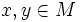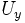# Locally homogeneous metric

This article defines a property that makes sense for a Riemannian metric over a differential manifold

## Definition

### Given data

A differential manifold$M$ equipped with a Riemannian metric$g$.

### Definition part$g$ is said to be locally homogeneous if for any$x,y \in M$ we can find neighbourhoods$U_x$ and$U_y$ of those and a Riemannian isometry between$U_x$ and$U_y$ that takes$x$ to$y$.